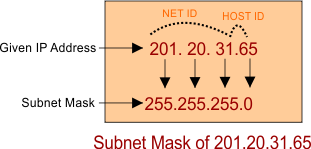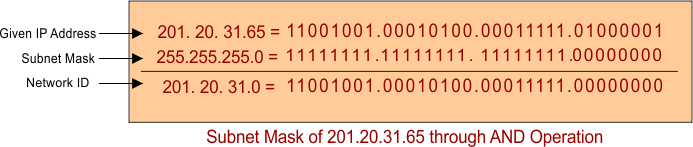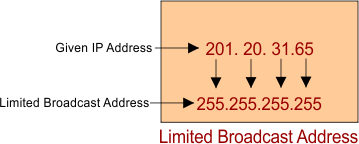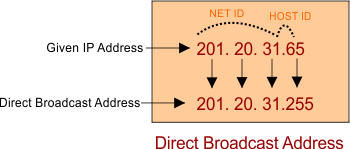Select Page

# Questions of Classful IP Addressing

Question is that if given IP address = 201.20.31.65 then find out the following parts

### Part 1: Find the Class of given IP?

Solution: As the first Octet of given IP address exist in between the range of Class C (192 – 223). So, given IP belongs to class C.

### Part 2: Find the Subnet Mask of given IP?

Solution: After checking the class of given IP. Subnet mask can be found by putting all octet-bits of network ID to “255” and Host bits to “0”. So, Class C subnet mask is 255.255.255.0.### Part 3: Find the Network ID?

Solution: To find network ID, Perform AND operation of given IP with Subnet mask in binary. It will provide the network ID where that particular IP exist. So network ID will be 201.20.31.0.### Part 4: Find First and Last IP address of given Network IP?

Solution: We know that there are two IP’s of each network are reserved. As the Network ID is 201.20.31.0. So, the first IP address will be 201.20.31.0 which is reserved for network identification. And Last IP address is 201.20.31.255 which is reserved for direct broadcasting.

### Part 5: Find first and Last Host ID of given Network IP?

Solution: Due to reservation of two Hosts, of Network ID is 201.20.31.0. So, the first host IP address will be 201.20.31.1 and Last host IP address is 201.20.31.254.Solution: By replacing the Host-bits-octets to 255 of given IP is called direct broadcast IP addressing. So, direct broadcast IP addressing = 201.20.31.255.Help Other’s By Sharing…